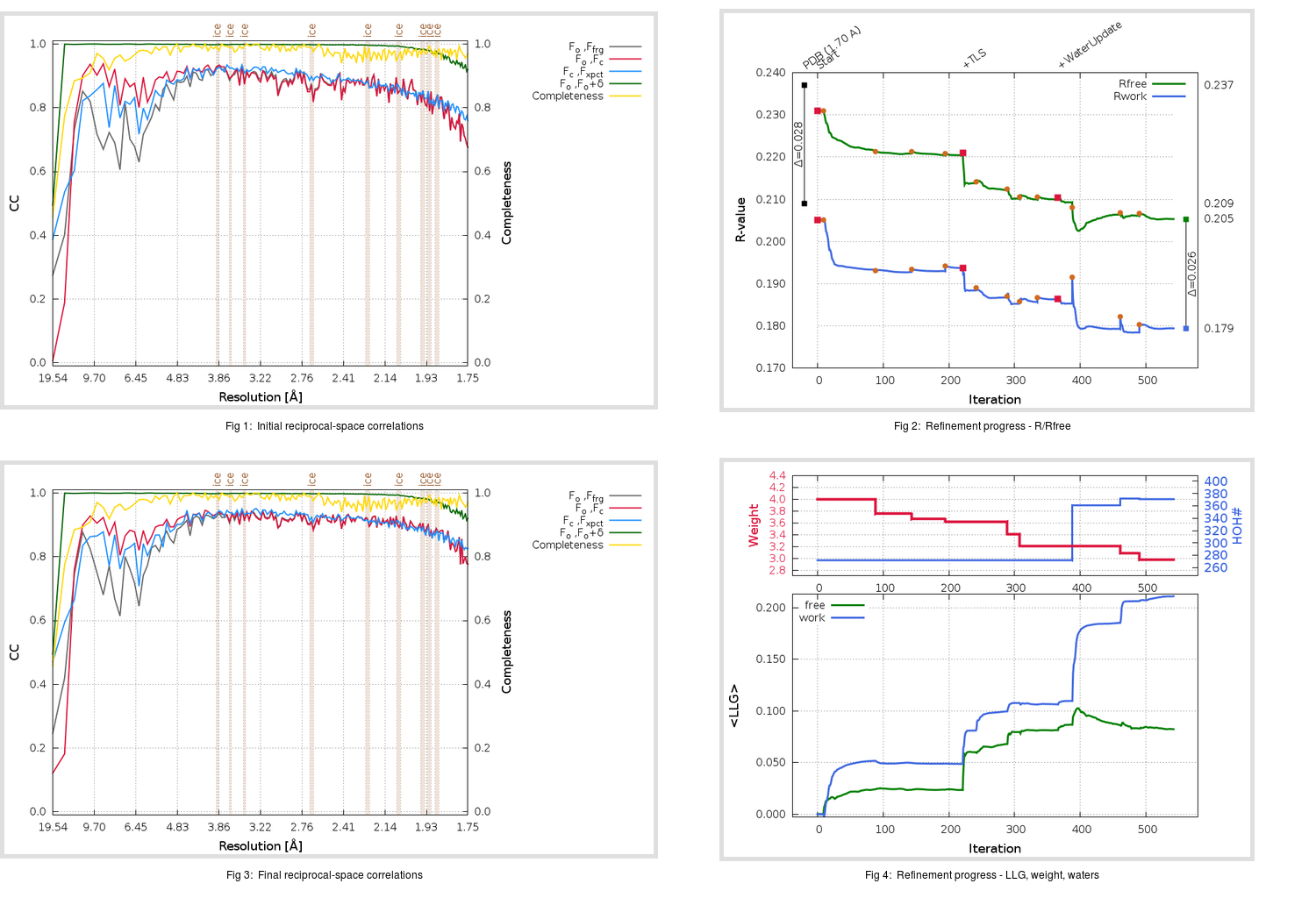Content:

```    Diffraction limits & principal axes of ellipsoid fitted to diffraction cut-off surface:
1.742         1.0000   0.0000   0.0000       a*
1.725         0.0000   1.0000   0.0000       b*
1.717         0.0000   0.0000   1.0000       c*
```

## Deposited

` `
 Date deposited Date data collection Resolution R, Rfree 20050304 20020615 1.70 0.2060 0.2370

Molprobity (CCP4 7.0 version) summary:

```Ramachandran outliers =   0.00 %
favored =  98.20 %
Rotamer outliers      =   0.34 %
C-beta deviations     =     0
Clashscore            =   3.56
RMS(bonds)            =   0.0042
RMS(angles)           =   1.10
MolProbity score      =   1.15
Resolution            =   1.70
R-work                =   0.2060
R-free                =   0.2370
```

```Number of waters      =   272

<B> (all atoms) =   24.08 ( sd =    8.45 ) for       3023 non-hydrogen atoms
<B>   (protein) =   23.34 ( sd =    8.13 ) for       2744 non-hydrogen atoms
<B>     (water) =   31.41 ( sd =    7.98 ) for        272 non-hydrogen atoms
<B>    (others) =   25.20 ( sd =    6.13 ) for          7 non-hydrogen atoms

B min/max       (all non-hydrogen atoms) =    9.35 /   53.26
B min/max   (protein non-hydrogen atoms) =    9.35 /   51.01
B min/max     (water non-hydrogen atoms) =   13.83 /   53.26
B min/max     (other non-hydrogen atoms) =   12.95 /   29.91
```

## BUSTER (re-)refinement

` `

Molprobity (CCP4 7.0 version) summary:

```Ramachandran outliers =   0.00 %
favored =  98.80 %
Rotamer outliers      =   0.68 %
C-beta deviations     =     0
Clashscore            =   0.94
RMS(bonds)            =   0.0127
RMS(angles)           =   1.38
MolProbity score      =   0.78
Resolution            =   1.75
R-work                =   0.1794
R-free                =   0.2053
```

```Number of waters      =   371

<B> (all atoms) =   26.83 ( sd =   10.18 ) for       3122 non-hydrogen atoms
<B>   (protein) =   25.28 ( sd =    8.93 ) for       2744 non-hydrogen atoms
<B>     (water) =   38.06 ( sd =   11.46 ) for        371 non-hydrogen atoms
<B>    (others) =   27.61 ( sd =    6.26 ) for          7 non-hydrogen atoms

B min/max       (all non-hydrogen atoms) =   12.84 /   81.94
B min/max   (protein non-hydrogen atoms) =   12.84 /   62.83
B min/max     (water non-hydrogen atoms) =   16.81 /   81.94
B min/max     (other non-hydrogen atoms) =   15.28 /   31.75
```

Refinement progression:Results:

` `
 File Remark 1Z1L_aB_refine.01_03_refine.pdb.gz exact refinement commands are in header 1Z1L_aB_refine.01_03_refine.mtz.gz including original deposited data and several re-refinement map coefficients 1Z1L_aB_refine.01_03_BUSTER_model.cif.gz including any non-standard compound restraints 1Z1L_aB_refine.01_03_BUSTER_refln.cif.gz